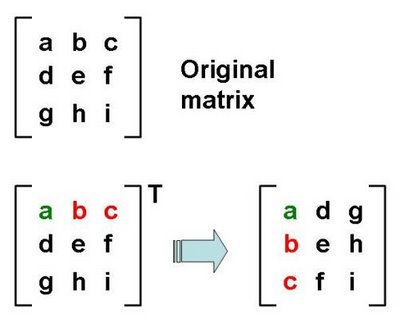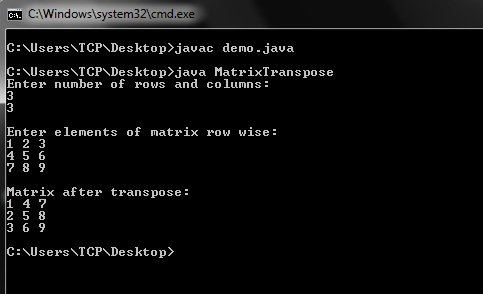# Java Program to Find Transpose of Matrix

Transpose of a matrix is a new matrix whose columns are the rows of original matrix and rows are the columns of original matrix. Take below example for transpose of matrix of order 3×3.Image Source

Below I have shared java program that will find transpose of matrix of any order. If you find any difficulty to understand then ask your queries in the comment section.

## Java Program to Find Transpose of Matrix### 1 thought on “Java Program to Find Transpose of Matrix”

1.